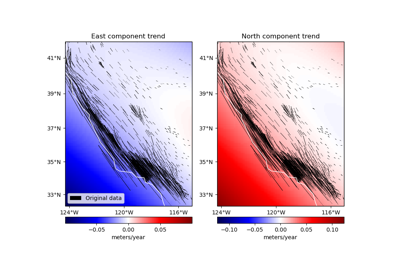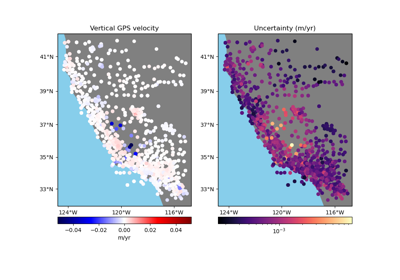# verde.variance_to_weights¶

verde.variance_to_weights(variance, tol=1e-15, dtype='float64')[source]

Converts data variances to weights for gridding.

Weights are defined as the inverse of the variance, scaled to the range [0, 1], i.e. variance.min()/variance.

Any variance that is smaller than tol will automatically receive a weight of 1 to avoid zero division or blown up weights.

Parameters
• variance (array or tuple of arrays) – An array with the variance of each point. If there are multiple arrays in a tuple, will calculated weights for each of them separately. Can have NaNs but they will be converted to zeros and therefore receive a weight of 1.

• tol (float) – The tolerance, or cutoff threshold, for small variances.

• dtype (str or numpy dtype) – The type of the output weights array.

Returns

weights (array or tuple of arrays) – Data weights in the range [0, 1] with the same shape as variance. If more than one variance array was provided, then this will be a tuple with the weights corresponding to each variance array.

Examples

>>> print(variance_to_weights([0, 2, 0.2, 1e-16]))
[1.  0.1 1.  1. ]
>>> print(variance_to_weights([0, 0, 0, 0]))
[1. 1. 1. 1.]
>>> for w  in variance_to_weights(([0, 1, 10], [2, 4.0, 8])):
...     print(w)
[1.  1.  0.1]
[1.   0.5  0.25]


## Examples using verde.variance_to_weights¶